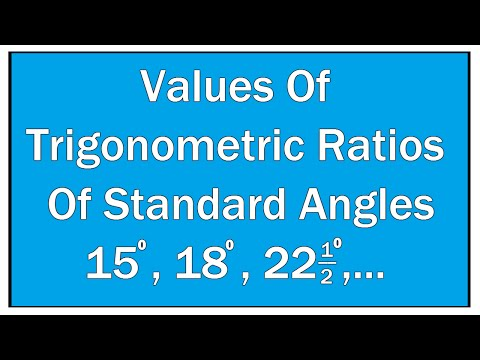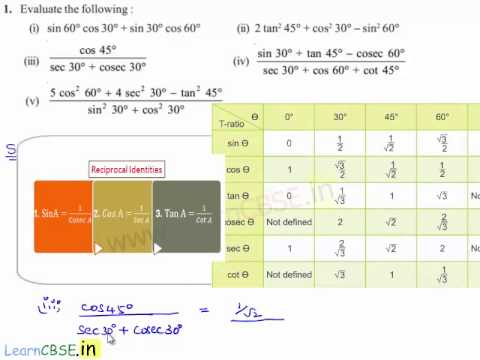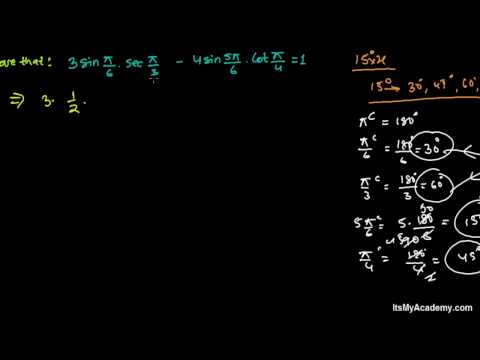## Trigonometric Ratios of Some Standard Angles

Subject: Optional Mathematics

#### Overview

Different angles have a different value with various trigonometric ratios. We shall consider 0°, 30°, 45° and 90° as the standard angles and we shall learn their values here. In this unit, we shall verify the values of 0°, 30°, 45° and 90° using geometrical proofs. Different angles have a different value with various trigonometric ratios. We shall consider 0°, 30°, 45° and 90° as the standard angles and we shall learn their values here. In this unit, we shall verify the values of 0°, 30°, 45° and 90° using geometrical proofs.

##### Trigonometric Ratios of Some Standard Angles

Different angles have a different value with various trigonometric ratios. We shall consider 0°, 30°, 45° and 90° as the standard angles and we shall learn their values here. In this unit, we shall verify the values of 0°, 30°, 45° and 90° using geometrical proofs.

Trigonometrical Ratio of 45°

Let ABC be a right-angledisosceles trianglewhere $\angle$B = 90° and $\angle$A = $\angle$C = 45°

Also let BC = AC = $\alpha$.

We know, in right angled $\triangle$ABC

h2 = p2 + b2

or, (AC)2 = (AB)2 + (BC)2

or, (AC)2 = $\alpha$2 + $\alpha$2

or, (AC)2 = 2 $\alpha$2

∴ AC = $\alpha$$\sqrt{2}$

Taking $\angle$C as the reference angle, we get,

sin 45 = $\frac{AB}{AC}$= $\frac{α}{α\sqrt{2}}$ = $\frac{1}{\sqrt{2}}$

cos 45 = $\frac{BC}{AC}$= $\frac{α}{α\sqrt{2}}$ = $\frac{1}{\sqrt{2}}$

tan 45 = $\frac{AB}{BC}$= $\frac{α}{α}$ = 1

cosec 45 = $\frac{AC}{AB}$= $\frac{α\sqrt{2}}{α}$ = $\sqrt{2}$

sec 45 = $\frac{AC}{AB}$= $\frac{α\sqrt{2}}{α}$ =$\sqrt{2}$

cot 45 = $\frac{BC}{AB}$ = $\frac{α}{α}$ = 1

Let ABC be an equilateral triangle where$\angle$A = $\angle$B = $\angle$C = 60°

and AB = BC = CA = 2$\alpha$

Now, let's draw AD perpendicular to BC so that,

BD = DC = a and $\angle$BAD = $\angle$DAC = 30°

Now, in right angled $\triangle$ADC,

or, (AD)2 = (AC)2 + (DC)2

= (2α)2 - (α)2

= 4$\alpha$2 - $\alpha$2 = 3$\alpha$2

$\therefore$ AD = $\alpha$$\sqrt{3}$

Now, to find the trigonometric ratio for 60°, lets take $\angle$C = 60° as the reference angle, we get,

sin 60° = $\frac{AD}{AC}$ = $\frac{\alpha\sqrt{3}}{2α}$ = $\frac{\sqrt{3}}{2}$

cos 60° = $\frac{DC}{AC}$= $\frac{α}{2α}$ =$\frac{1}{2}$

tan 60° = $\frac{AD}{DC}$=$\frac{\alpha\sqrt{3}}{2α}$ = $\sqrt{3}$

cosec 60° = $\frac{AC}{AD}$= $\frac{2α}{\alpha\sqrt{3}}$ = $\frac{2}{\sqrt{3}}$

sec 60° = $\frac{AC}{DC}$= $\frac{2α}{α}$ = 2

cot 60° = $\frac{DC}{AD}$= $\frac{α}{\alpha\sqrt{3}}$ = $\frac{1}{\sqrt{3}}$

Now, to find out the trigonometric ratio for 30° , let's take $\angle$DAC = 30° as the reference angle, we get,

sin 30° =$\frac{DC}{AC}$= $\frac{α}{2α}$ =$\frac{1}{2}$

cos 30° = $\frac{AD}{AC}$ = $\frac{\alpha\sqrt{3}}{2α}$ = $\frac{\sqrt{3}}{2}$

tan 30° =$\frac{DC}{AD}$= $\frac{α}{\alpha\sqrt{3}}$ = $\frac{1}{\sqrt{3}}$

cosec 30° =$\frac{AC}{DC}$= $\frac{2α}{α}$ = 2

sec 30° = $\frac{AC}{AD}$= $\frac{2α}{\alpha\sqrt{3}}$ = $\frac{2}{\sqrt{3}}$

cot 30° =$\frac{AD}{DC}$=$\frac{\alpha\sqrt{3}}{2α}$ = $\sqrt{3}$

Trigonometrical Ratio of 0°

Let ABC be a right angled triangle where $\angle$B = 90° and $\angle$C = $\theta$.

If $\theta$ tends to 0°

i.e $\theta$→0°, AC coincides with BC.

or, AC $\approx$BC

so, AC= BC = a (say)

Now, using pythagoras theorem

h2= p2+ b2

or, (AC)2=(AB)2+ (BC)2

or, a2 = (AB)2 + a2

or, (AB)2 = a2 - a2 = 0

$\therefore$ AB = 0

Now, taking right angled $\triangle$ABC

sin 0° = $\frac{p}{h}$ =$\frac{AB}{AC}$ =$\frac{0}{a}$ = 0

cos 0° =$\frac{b}{h}$ = $\frac{BC}{AC}$ = $\frac{a}{a}$ = 1

tan 0° =$\frac{p}{b}$ = $\frac{AB}{BC}$ = $\frac{0}{a}$ = 0

cosec 0° =$\frac{h}{p}$ = $\frac{AC}{AB}$ = $\frac{a}{0}$ = $\infty$

sec 0° =$\frac{h}{b}$ = $\frac{AC}{BC}$ = $\frac{a}{a}$ = 1

cot 0° =$\frac{b}{p}$ = $\frac{BC}{AB}$ = $\frac{a}{0}$ = $\infty$

Trigonometric Ratio of 90°

Let ABC be a right angled triangle where $\angle$B = 90° and $\angle$C = $\theta$ be the reference angle.

If $\theta$ tends to 90°

i.e $\theta$→90°, AC coincides with BC.

or, AC $\approx$AB

so, AC= AB = a (say)

Now, using pythagoras theorem

h2= p2+ b2

or, (AC)2=(AB)2+ (BC)2

or, a2 = (BC)2 + a2

or, (BC)2 = a2 - a2 = 0

$\therefore$ BC = 0

Now, taking right angled $\triangle$ABC

sin 90° = $\frac{p}{h}$ =$\frac{AB}{AC}$ =$\frac{a}{a}$ =1

cos 90° =$\frac{b}{h}$ = $\frac{BC}{AC}$ = $\frac{0}{a}$ = 0

tan 90° =$\frac{p}{b}$ = $\frac{AB}{BC}$ = $\frac{a}{0}$ =$\infty$

cosec 90° =$\frac{h}{p}$ = $\frac{AC}{AB}$ = $\frac{a}{a}$ =1

sec 90° =$\frac{h}{b}$ = $\frac{AC}{BC}$ = $\frac{a}{0}$ = $\infty$

cot 90° =$\frac{b}{p}$ = $\frac{BC}{AB}$ = $\frac{0}{a}$ =0

#### Complementary Angles

Two angles are Complementary when they add up to 90 degrees.

Let'a see the following example,

What is the angle added to 60° and 90°?

Let the angle be x.

Then, x + 60° = 90°

or, x = 90° - 60° = 30°

Hence, 30° and 60° when added together gives us 90°, So, 30° and 60° are called complements of each other.

The angles are said to be complementary if the sum of the angles is 90°.

Complementary Angles in Trigonometry

Let ABC be a right angledtriangle where $\angle$ABC = 90° and $\angle$ACB = $\theta$

Now, we know,

$\angle$ACB +$\angle$ABC +$\angle$BAC = 180° (sum of angles of a $\triangle$)

or, $\theta$ + 90° + $\angle$BAC = 180°

or, $\angle$BAC = 180° - 90° - $\theta$ = 90° - $\theta$

So, $\angle$ACB and$\angle$CAB are complementary angles.

Now, taking$\angle$A = 90° - $\theta$ as the reference angle, we get,

BC = perpendicular (p)

AC = hypotenuse (h)

AB = base (b)

So,

sin(90° - $\theta$) = $\frac{p}{h}$ =$\frac{BC}{AC}$ = cos$\theta$ (For reference angle $\theta$)

cos (90° - $\theta$) = $\frac{b}{h}$ =$\frac{AB}{AC}$ = sin$\theta$

tan(90° - $\theta$) =$\frac{p}{h}$ =$\frac{BC}{AB}$ = cot$\theta$

cot(90° - $\theta$) =$\frac{b}{p}$ =$\frac{AB}{BC}$ = tan$\theta$

sec(90° - $\theta$) =$\frac{h}{b}$ =$\frac{AC}{AB}$ = cosec$\theta$

cosec(90° - $\theta$) =$\frac{h}{p}$ =$\frac{AB}{BC}$ = sec$\theta$

Hence,

sin(90° - $\theta$) = cos$\theta$

cos(90° - $\theta$) = sin$\theta$

tan(90° - $\theta$) =cot$\theta$

cot(90° - $\theta$) =tan$\theta$

sec(90° - $\theta$) = cosec$\theta$

cosec(90° - $\theta$) =sec$\theta$

##### Things to remember
• h2 = p2 + b2
• Different angles have a different value with various trigonometric ratios. We shall consider 0°, 30°, 45° and 90° as the standard angles and we shall learn their values here. In this unit, we shall verify the values of 0°, 30°, 45° and 90° using geometrical proofs.
• It includes every relationship which established among the people.
• There can be more than one community in a society. Community smaller than society.
• It is a network of social relationships which cannot see or touched.
• common interests and common objectives are not necessary for society.
##### Videos for Trigonometric Ratios of Some Standard Angles##### Find The Values Of Trigonometric Ratios Of Standard angles 15°,18°,.... / Maths Trigonometry##### Solving Problems on Trigonometric Ratio of Standard Angle algebraically##### Tricks to Solve Trigonometry Ratios of Standard Angles Questions##### Trigonometric ratios of standard angles

soln: L.H.S = sin4200 cos 3900 + cos (-3000) sin (-3300)

= sin4200 cos3900 + cos3000 (-sin3300)

= sin4200 cos3900 - cos3000 sin3300

= sin (3600 + 600) cos (3600 + 300) - cos (3600-60o) sin(3600-300)

= sin600 cos300 cos600 (-sin 300) = sin600 cos300 + cos600sin300

= $\frac{(\sqrt{3})}{2}$×$\frac{(\sqrt{3})}{2}$+$\frac{1}{2}$×$\frac{1}{2}$=$\frac{3}{4}$+$\frac{1}{4}$=$\frac{3+1}{4}$=$\frac{4}{4}$=1= R.H.S. proved.

Given that A = 30°

Then, LHS = sin3A

= sin3 × 30°

= sin90°

= 1

RHS = 3sinA - 4sin3A

= 3sin30° - 4(sin30°)3

= 3$\frac{1}{2}$ - 4($\frac{1}{2}$)3

= $\frac{3}{2}$ - 4 $\frac{1}{8}$

= $\frac{3}{2}$ - $\frac{1}{2}$

= $\frac{2}{2}$

= 1

$\therefore$ LHS = RHS verified.

Solution

LHS = cos 60

= $\frac{1}{2}$

RHS = cos230° - sin230°

($\frac{\sqrt(3)}{2}$)2 - ($\frac{1}{2}$)2

$\frac{3}{4}$ - $\frac{1}{4}$

$\frac{2}{4}$

$\frac{1}{2}$

$\therefore$ LHS = RHS proved.

Solution

Here, x×sin30° cos245° = $\frac{cot^230° sec60° tan45°}{cosec^245°×cosec30°}$

or, x×$\frac{1}{2}$×($\frac{1}{\sqrt(2)}$)2 = $\frac{(\sqrt(3)^2×2×1)}{(\sqrt(2)^ 2×2}$

or, x = $\frac{3×2×2×2}{2×2}$ = 6

Solution

Here, cot 3$\theta$ = tan 7$\theta$

or, cot 3$\theta$ = cot (90° -7$\theta$) [cot(90° - $\theta) = tan\theta$]

or, 3$\theta$ = 90° - 7$\theta$

or,10$\theta$ = 90°

$\therefore$ $\theta$ = 9°

Solution

Here, In ABC

B = 90° (Since Ac is the hypotenuse.)

So, using Pythagoras theorem

(AC)2 = (AB)2 + (BC)2

= (1)2 +($\sqrt(3)$)2

= 1 + 3 = 4
or, AC = 2 = b

Now, sinA =$\frac{BC}{AC}$

or, sinA = $\frac{\sqrt(3)}{2}$

or, sinA = sin 60°

$\therefore$ A = 60°

Again,

$\angle$A + $\angle$B + $\angle$C = 180°

or, 60° + 90° + $\angle$C = 180°

C = 180° - 150° = 30°

Hence, b = 2, A = 60° and C = 30°

Solution

sec2$\frac{π}{4}$ sec2$\frac{π}{3}$(cosec$\frac{π}{6}$ - cosec$\frac{π}{2}$)

= sec2 $\frac{180°}{4}$ × sec2$\frac{180°}{3}$ (cosec$\frac{180°}{6}$ - cosec$\frac{180°}{2}$)

= sec245° × sec260° (cosec30° - cosec90°)

= ($\sqrt(2)$)2 × (2)2 (2-1)

= 2×4×1

= 8